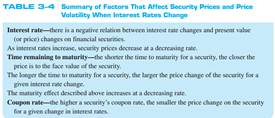### Create an Account

Home / Questions / A bond you are evaluating has a 10 percent coupon rate compounded semiannually a \$1,000 fa...

# A bond you are evaluating has a 10 percent coupon rate compounded semiannually a \$1,000 face value and is 10 years from maturity If the required rate of return on the bond is

A bond you are evaluating has a 10 percent coupon rate (compounded semiannually), a \$1,000 face value, and is 10 years from maturity. ( LG 3-4)

a. If the required rate of return on the bond is 6 percent, what is its fair present value?

b. If the required rate of return on the bond is 8 percent, what is its fair present value?

c. What do your answers to parts (a) and (b) say about the relation between required rates of return and fair values of bonds?

( LG 3-4)

As already discussed in this chapter and in Chapter 2 , the variability of financial security prices depends on interest rates and the characteristics of the security. Specifically, the factors that affect financial security prices include interest rate changes, the time remaining to maturity, and the coupon rate. We evaluate next the impact of each of these factors as they affect bond prices. The impact on equity prices is similar. Table 3–4 summarizes the major relationships we will be discussing.May 18 2020 View more View LessSubscribe To Get Solution